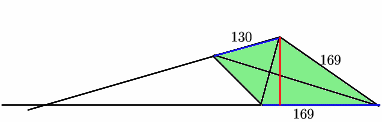#### You may also likeFour rods are hinged at their ends to form a convex quadrilateral. Investigate the different shapes that the quadrilateral can take. Be patient this problem may be slow to load.### Long Short

What can you say about the lengths of the sides of a quadrilateral whose vertices are on a unit circle?### Dividing the Field

A farmer has a field which is the shape of a trapezium as illustrated below. To increase his profits he wishes to grow two different crops. To do this he would like to divide the field into two trapeziums each of equal area. How could he do this?

# Lawnmower

##### Age 14 to 16Challenge Level

Tony Cardell, has sent in this solution, which gives the correct answer. We're still not quite convinced: how does he know that the strips are parallel to the longer side? If anyone can explain this, we'll add their explanation here.

We must find the maximum distance between a pair of opposite sides (it doesn't matter which since this is a kite). If we extend the two sides (coloured blue), the intersection is on the same side of the kite as the equilateral triangle, as shown. So the maximum distance between the two sides is the red line.Now look at the isosceles triangle part of the kite. One formula for the area of a triangle with sides a,b,c says that if s is the semi-perimeter, (a+b+c)/2, then the area is the square root of s(s-a)(s-b)(s-c). The isosceles triangle has sides 169, 169, 130, so s=234 and the area is 10140 square feet. But we also know that the area is 1/2 x base x height, so the height we want is 10140 x 2 / 169=120. So 120 foot-wide strips will be needed.